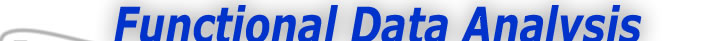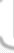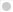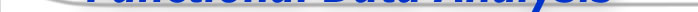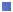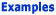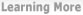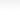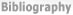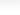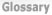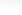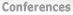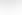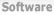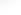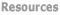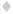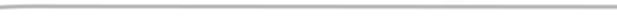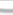BasisFunctions Goods -Smoothing with a fourth order roughness penalty -A first look at the seasonal variation Growth Weather
 Smoothing the Nondurable Goods Index Previous - 1 - 2 - Next: A first look at the seasonal variation Expertise: Intermediate Smoothing with a fourth order roughness penalty The process generating the data is essentially smooth since the amount of the data upon which it is based precludes abrupt changes in value. we can exploit this smoothness by making use of one or more derivatives in our explorations. In fact, we will find that both the rate of change or velocity and the curvature or acceleration of the index are useful, and this implies that we should have a smooth estimate of acceleration. We choose B-spline basis functions as our basis function system. Why? We don't use Fourier series here because we don't want to assume the stationarity of seasonal trend that is built into this basis. On the other hand, B-spline basis functions are sufficiently flexible to be able to capture both the long-term and seasonal trends that interest us. Moreover, we can ensure a smooth second derivative by specifying the order of the spline (the degree plus one of the piecewise polynomials from which a B-spline basis function is constructed) to be eight. A knot was placed at each data point. The fitting criterion iswhere D4x(t) means the fourth derivative of function x at time t. We liked the results for smoothing parameter λ = 10-11 since the data points were fit to our satisfaction and the second derivative curve was smooth. A bit of jargon: When the order of the spline is twice the order of the derivative being penalized, and a knot is placed at each data point, we call the fitted spline function a smoothing spline. And a computational note: This spline is defined by 977 basis functions, namely the number of interior knots plus the order. Without some special computational technology, solving the set of linear equations of order 977 would challenge the resources most small computers and try the patience of the data analyst. In Matlab, however, there is the possibility of doing computation in sparse matrix mode, and most of the elements in the coefficient matrix are zero because of its banded structure. In S-PLUS, on the other hand, the computation had to be coded in C to take advantage of the banded structure, and this code is called by S-PLUS function smooth.Pspline. Previous - 1 - 2 - Next: A first look at the seasonal variation